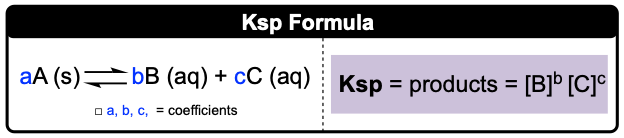Clutch Prep is now a part of Pearson
Ch.16 - Aqueous Equilibrium WorksheetSee all chapters

# Solubility Product Constant: Ksp

See all sections
Sections
Intro to Buffers
Henderson-Hasselbalch Equation
Intro to Acid-Base Titration Curves
Strong Titrate-Strong Titrant Curves
Weak Titrate-Strong Titrant Curves
Acid-Base Indicators
Titrations: Weak Acid-Strong Base
Titrations: Weak Base-Strong Acid
Titrations: Strong Acid-Strong Base
Titrations: Diprotic & Polyprotic Buffers
Solubility Product Constant: Ksp
Ksp: Common Ion Effect
Precipitation: Ksp vs Q
Selective Precipitation
Complex Ions: Formation Constant

Solubility is the maximum amount of solid dissolved in a solvent. Ksp measures the solubilities of solid ionic compounds in a solvent at equilibrium.

###### Ksp

Concept #1: Solubility Product Constant (Ksp)

Generally, the larger the Ksp, the more soluble the solid is.

Example #1: Given the following ionic compounds, which will have the highest [OH-] concentration? Hint: which is most soluble in water?

a) Fe(OH)2 Ksp = 4.87 x 10-17               b) Pb(OH)2 Ksp = 1.43 x 10-20

c) Mg(OH)Ksp = 2.06 x 10-13               d) Sn(OH)2 Ksp = 5.45 x 10-27

Concept #2: Ksp CalculationsExample #2: PbF2 is a white solid and has diverse applications in pharmaceuticals, metallurgy, and technology. If the concentration of lead (II) fluoride is 4.2 M with a Ksp = 3.6 x 10-8, calculate the molar solubility of this solid at 25ºC.

Practice: Solubility of Sn(OH)2 was found to be 1.11 x 10-9 M; calculate Ksp of this compound.

Practice: If a saturated solution of Ag2CO3 contains 2.56 × 104 M of Ag+ ions, determine its solubility product constant.

Practice: What is the solubility of CN ions in a solution of 5.5 M Hg2(CN)2, with a Ksp of 5.0 × 1040?